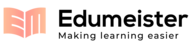class m_sorted_list:
def __init__(self, m_sorted):
self.__sorted = m_sorted
if self.__sorted == False:
self.__dlist.append(item)
else:
temp = self.__dlist.search_larger(item)
if (temp == -1):
temp = self.__dlist.get_size()
self.__dlist.insert(temp,item)
def pop(self):
if self.__sorted == False:
return self.__dlist.pop(0)
else:
return self.__dlist.pop1()
def search(self, item):
found = self.__dlist.search(item)
if (found):
inde = self.__dlist.index(item)
return found,inde
if self.__sorted == False:
return found, -1
else:
return found , self.__dlist.search_larger(item)
def change_sorted(self):
if self.__sorted == False:
raise Exception("I don’t know how to sort a doubly linked
list yet")
else:
self.__sorted = False
def get_size(self):
return self.__dlist.get_size()
def get_item(self, pos):
if pos < 0 or pos >= self.__dlist.get_size():
raise Exception("No negatives here")
else:
return self.__dlist.get_item(pos)
def __str__(self):
return str(self.__dlist)
def test():
sor_list = m_sorted_list(True)
is_pass = (sor_list.get_size() == 0)
assert is_pass == True, "fail the test"
is_pass = (str(sor_list) == "1 3 4 7 8")
assert is_pass == True, "fail the test"
is_pass = (sor_list.get_size() == 5)
assert is_pass == True, "fail the test"
is_pass = (sor_list.pop() == 8)
assert is_pass == True, "fail the test"
is_pass = (sor_list.pop() == 7)
assert is_pass == True, "fail the test"
is_pass = (str(sor_list) == "1 3 4")
assert is_pass == True, "fail the test"
a = sor_list.search(2)
is_pass = (a == False and a == 1)
assert is_pass == True, "fail the test"
a = sor_list.search(3)
is_pass = (a == True and a == 1)
assert is_pass == True, "fail the test"
a = sor_list.search(7)
is_pass = (a == False and a == -1)
assert is_pass == True, "fail the test"
is_pass = (sor_list.get_size() == 3)
assert is_pass == True, "fail the test"
is_pass = (sor_list.get_item(2) == 4)
assert is_pass == True, "fail the test"
sor_list.change_sorted()
is_pass = (str(sor_list) == "1 3 4 1")
assert is_pass == True, "fail the test"
is_pass = (sor_list.get_size() == 4)
assert is_pass == True, "fail the test"
is_pass = (sor_list.pop() == 1)
assert is_pass == True, "fail the test"
is_pass = (sor_list.pop() == 3)
assert is_pass == True, "fail the test"
is_pass = (str(sor_list) == "4 1 7 6")
assert is_pass == True, "fail the test"
a = sor_list.search(2)
is_pass = (a == False and a == -1)
assert is_pass == True, "fail the test"
a = sor_list.search(7)
is_pass = (a == True and a == 2)
assert is_pass == True, "fail the test"
a = sor_list.search(8)
is_pass = (a == False and a == -1)
assert is_pass == True, "fail the test"
is_pass = (sor_list.get_size() == 4)
assert is_pass == True, "fail the test"
is_pass = (sor_list.get_item(2) == 7)
assert is_pass == True, "fail the test"
sor_list2 = m_sorted_list(False)
is_pass = (sor_list2.get_size() == 0)
assert is_pass == True, "fail the test"
is_pass = (str(sor_list2) == "4 3 8 7 1")
assert is_pass == True, "fail the test"
is_pass = (sor_list2.get_size() == 5)
assert is_pass == True, "fail the test"
is_pass = (sor_list2.pop() == 4)
assert is_pass == True, "fail the test"
is_pass = (sor_list2.pop() == 3)
assert is_pass == True, "fail the test"
is_pass = (str(sor_list2) == "8 7 1")
assert is_pass == True, "fail the test"
a = sor_list2.search(2)
is_pass = (a == False and a == -1)
assert is_pass == True, "fail the test"
a = sor_list2.search(7)
is_pass = (a == True and a == 1)
assert is_pass == True, "fail the test"
is_pass = (sor_list2.get_size() == 3)
assert is_pass == True, "fail the test"
is_pass = (sor_list2.get_item(2) == 1)
assert is_pass == True, "fail the test"
try:
sor_list2.change_sorted()
except Exception as e:
is_pass = True
else:
is_pass = False
assert is_pass == True, "fail the test"
is_pass = (str(sor_list2) == "8 7 1 3 2")
assert is_pass == True, "fail the test"
is_pass = (sor_list2.get_size() == 5)
assert is_pass == True, "fail the test"
is_pass = (sor_list2.pop() == 8)
assert is_pass == True, "fail the test"
is_pass = (sor_list2.pop() == 7)
assert is_pass == True, "fail the test"
is_pass = (str(sor_list2) == "1 3 2")
assert is_pass == True, "fail the test"
if is_pass == True:
print ("=========== Congratulations! Your have finished exercise
2! ============")
if __name__ == '__main__':
test()